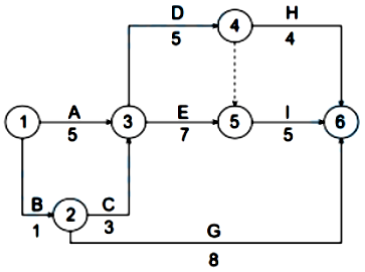165:002023年6月4日《建设施工管理》真题（1天考3科）（精编）

1
(单项选择题)

• A.

10

• B.

15

• C.

20

• D.

30

• A
• B
• C
• D

2
(单项选择题)

• A.

施工预算

• B.

施工图预算书

• C.

签约合同价

• D.

责任成本目标

• A
• B
• C
• D

3
(单项选择题)

• A.

焊缝超声波探伤

• B.

钢筋强度测定

• C.

门窗口的对角线检查

• D.

混凝土外观检查

• A
• B
• C
• D

4
(单项选择题)

• A.

设置质量控制点

• B.

对质量活动进行监督

• C.

对质量活动结果进行评价

• D.

落实质量责任

• A
• B
• C
• D

5
(单项选择题)

• A.

混凝土模板

• B.

冬雨期施工

• C.

垂直运输

• D.

室内空气污染测试

• A
• B
• C
• D

6
(单项选择题)

• A.

项目级评价每年由企业安全生产与环境管理人员组织，每年进行一次

• B.

企业最高管理者可根据合规性评价结果结合本企业实际情况提出改进要求

• C.

公司级评价由项目经理组织，每半年进行一次

• D.

合规性评价应形成重要的记录和证据并作管理评审的输出结果

• A
• B
• C
• D

7
(单项选择题)

• A.

质量保证金保函

• B.

等价抵押物

• C.

一定比例的工程款

• D.

双方约定的其他方式

• A
• B
• C
• D

8
(单项选择题)

• A.

满足顾客要求

• B.

全员积极参与

• C.

领导作用

• D.

持续改进

• A
• B
• C
• D

9
(单项选择题)

• A.

施工单位法定代表人

• B.

项目经理

• C.

项目总工程师

• D.

安全管理责任人

• A
• B
• C
• D

10
(单项选择题)

• A.

伪造事故现场

• B.

迟报或者漏报事故

• C.

干涉事故调查工作

• D.

维护负有事故责任的人员

• A
• B
• C
• D

11
(单项选择题)

• A.

不利于业主的投资控制

• B.

与平行发承包模式相比，组织协调工作量大

• C.

建设周期长，对项目总进度控制不利

• D.

施工总承包合同一般采用成本加酬金合同

• A
• B
• C
• D

12
(单项选择题)

• A.

确定计划期内的人工需求

• B.

论证项目的进度目标

• C.

确定施工作业的具体安排

• D.

施工动态控制的依据

• A
• B
• C
• D

13
(单项选择题)

• A.

为完成工程所需要追加的额外工作

• B.

取消承包人合同中的某项工作，转由第三人实施

• C.

改变合同工程的标高

• D.

改变合同中某一项工作的质量标准

• A
• B
• C
• D

14
(单项选择题)

• A.

7

• B.

28

• C.

14

• D.

36

• A
• B
• C
• D

15
(单项选择题)

• A.

风险减轻

• B.

风险自留

• C.

风险转移

• D.

风险规避

• A
• B
• C
• D

16
(单项选择题)• A.

①－②－③－⑤－⑥

• B.

①－③－④－⑥

• C.

①－③－⑤－⑥

• D.

①－②－⑥

• A
• B
• C
• D

17
(单项选择题)

• A.

组织或参与评价项目管理绩效

• B.

主持确定大宗资源的供应单位

• C.

建立各类专业管理制度并组织实施

• D.

主持项目管理机构工作

• A
• B
• C
• D

18
(单项选择题)

• A.

3329

• B.

3396

• C.

3429

• D.

3433

• A
• B
• C
• D

19
(单项选择题)

• A.

政府建设主管部门

• B.

施工合同约定

• C.

国家法律

• D.

质量管理体系

• A
• B
• C
• D

20
(单项选择题)

• A.

12

• B.

24

• C.

48

• D.

2

• A
• B
• C
• D

21
(单项选择题)

• A.

业主改变设计方案的风险

• B.

自然环境变化的风险

• C.

对环境调查和预测的风险

• D.

合同所依据法律环境变化的风险

• A
• B
• C
• D

22
(单项选择题)

• A.

材料费

• B.

工程建设其他费

• C.

施工机具使用费

• D.

企业管理费

• A
• B
• C
• D

23
(单项选择题)

• A.

质量手册

• B.

程序文件

• C.

质量计划

• D.

质量记录

• A
• B
• C
• D

24
(单项选择题)

• A.

一般事故

• B.

较大事故

• C.

特别重大事故

• D.

重大事故

• A
• B
• C
• D

25
(单项选择题)

• A.

耗用的材料费

• B.

检验试验费

• C.

施工企业管理费

• D.

临时设施摊销费

• A
• B
• C
• D

26
(单项选择题)

• A.

10

• B.

11

• C.

12

• D.

13

• A
• B
• C
• D

27
(单项选择题)

• A.

测定法

• B.

理论计算法

• C.

估算法

• D.

图纸计算法

• A
• B
• C
• D

28
(单项选择题)

• A.

工程施工不符合工程设计要求

• B.

工程施工不符合监理规范

• C.

工程施工不符合合同约定

• D.

工程施工不符合技术标准

• A
• B
• C
• D

29
(单项选择题)

• A.

1.67%

• B.

2.54%

• C.

9.23%

• D.

2.69%

• A
• B
• C
• D

30
(单项选择题)

• A.

招标文件和投标文件不一致的地方应以招标文件为准

• B.

合同约定不得违背招标、投标文件中关于工期、质量和造价的实质性内容

• C.

不实行招标的政府投资工程合同价款，发承包双方可以在合同中约定不执行清单规范

• D.

不实行招标的抢险、救灾工程合同价款应以施工图预算为基础

• A
• B
• C
• D

31
(单项选择题)

• A.

现场焊接条件和工艺

• B.

焊缝探伤结果

• C.

焊件材料质量

• D.

焊接钢结构形式

• A
• B
• C
• D

32
(单项选择题)

• A.

赔偿范围包括承包人在工地的财产损失

• B.

被保险人是项目法人和承包人以外的第二人

• C.

被保险人是项目法人和承包人

• D.

赔偿范围包括承包人在现场从事与工作有关的职工伤亡

• A
• B
• C
• D

33
(单项选择题)

• A.

对测量基准点及其书面资料的真实性、准确性和完整性负责

• B.

办理取得出入施工场地的专用和临时道路通行权

• C.

按工程需要提供夜间施工使用的照明

• D.

组织工程项目竣工验收

• A
• B
• C
• D

34
(单项选择题)

• A.

方法

• B.

环境

• C.

机械

• D.

• A
• B
• C
• D